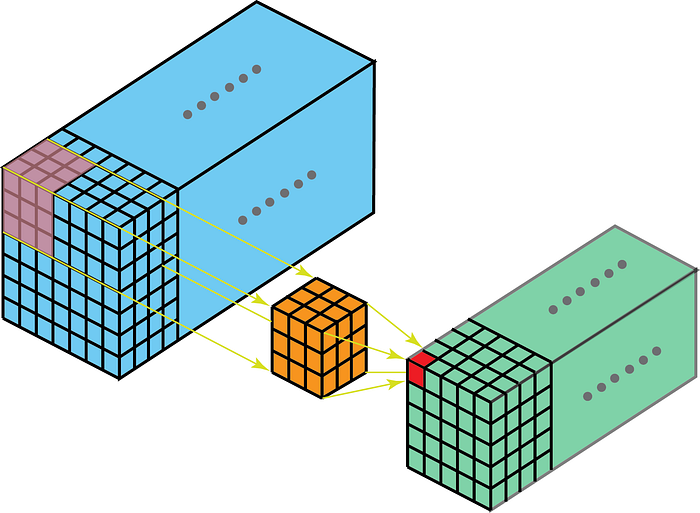# When should I use 3D convolutions?

I am new to convolutional neural networks, and I am learning 3D convolution. What I could understand is that 2D convolution gives us relationships between low-level features in the X-Y dimension, while the 3D convolution helps detect low-level features and relationships between them in all the 3 dimensions.

Consider a CNN employing 2D convolutional layers to recognize handwritten digits. If a digit, say 5, was written in different colors:Would a strictly 2D CNN perform poorly (since they belong to different channels in the z-dimension)?

Also, are there practical well-known neural nets that employ 3D convolution?

• 3D convolutions are commonly used for processing 3D images such as MRI scans. Jul 31, 2019 at 6:37
• Are there any publications on 3D Conv architectures? Jul 31, 2019 at 6:53
• @Shobhit given the answer by ashenoy, is there some part of your question that has not been answered yet? Aug 7, 2019 at 11:50

3D convolutions are used when you want to extract features in 3 dimensions or establish a relationship between 3 dimensions.

Essentially, it's the same as 2D convolutions, but the kernel movement is now 3-dimensional, causing a better capture of dependencies within the 3 dimensions and a difference in output dimensions post convolution.

The kernel of the 3d convolution will move in 3-dimensions if the kernel's depth is lesser than the feature map's depth.On the other hand, 2-D convolutions on 3-D data mean that the kernel will traverse in 2-D only. This happens when the feature map's depth is the same as the kernel's depth (channels).Some use cases for better understanding are

• MRI scans where the relationship between a stack of images is to be understood;

• low-level feature extractor for spatio-temporal data, like videos for gesture recognition, weather forecasting, etc. (3-D CNN's are used as low level feature extractors only over multiple short intervals, as 3D CNN's fail to capture long term spatio-temporal dependencies - for more on that check out ConvLSTM or an alternate perspective here.)

Most CNN models that learn from video data almost always have 3D CNN as a low level feature extractor.

In the example you have mentioned above regarding the number 5 - 2D convolutions would probably perform better, as you're treating every channel intensity as an aggregate of the information it holds, meaning the learning would almost be the same as it would on a black and white image. Using 3D convolution for this, on the other hand, would cause learning of relationships between the channels which do not exist in this case! (Also 3D convolutions on an image with depth 3 would require a very uncommon kernel to be used, especially for the use case)

3D convolutions should be used when you want to extract spatial features from your input on 3 dimensions. For computer vision, they are typically used on volumetric images, which are 3D.

Some examples are classifying 3D rendered images and medical image segmentation.